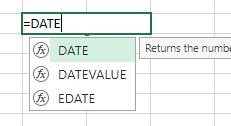## Excel – Calculate age with the DATEDIF function

Calculating age in a spreadsheet can be a painful process using many of the formulas floating around the web today. Dates act strangely in spreadsheets so care must be taken when working with them. Fortunately, calculating age can be done much easier using a single function in Excel called DATEDIF. The DATEDIF function will return the difference between two dates in days, months, or years. Using these units in combination can get you a variety of output options.DATEDIF is not in the list of functions

This function is a carry over from the Lotus 1-2-3 days and is not an official function supported in Excel. Therefore, Excel won’t help you with hints when you type the function.

Google Sheets has this function in their core list of functions. There is no discussion of the MD error (see below) with Sheets.

## Syntax

`=DATEDIF(start_date,end_date,unit)`

`start_date` Date at which to start the calculation

`end_date` Date at which to end the calculation

`unit` Type of output. Choices are “Y”,”M”,”D”,”YM”,”YD”, or “MD”.

• Y – Number of whole years elapsed between start and end dates
• YM – Number of months elapsed after the number of years shown with the “Y” unit. Will not exceed 11.
• YD – Number of days elapsed after the number of years shown with the “Y” unit. No matter how many days after the last year, starts counting after end of last full year and is never over 364.
• M – Number of whole months elapsed between start and end dates
• MD – Number of days elapsed after the number of months shown with the “M” or “YM” unit. Can’t go higher than 30.
• Beware that the MD option can cause errors.
• D – Number of whole days elapsed between start and end dates

## Examples

Let’s say that you have three columns of data. As long as you have start and end dates, you are ready to go.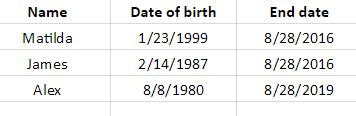Columns for name, start date, and end date

Now add 4 columns to the right for the DATEDIF formula with “Y”, “YM”, “YD”, and a column with them joined together. The Y, YM and MD are used as the “unit” in each respective column. The “Y” column is showing the person’s age in years. The “YM” is showing the number of months that have elapsed since the last year shown. The “MD” column is showing the number of days that have elapsed since the last whole month.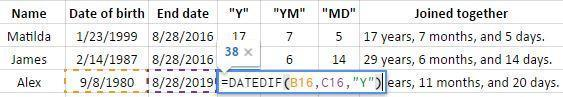Y, YM, and MD units added

There are other types of units as well. This next example uses M and MD. Note that the number of months goes over twelve since the years are not in the formula’s output. The column using MD as the unit is the same calculation as above.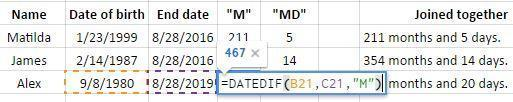M and MD units

This last example uses just the D unit. If you are just using this function to calculate days, it would be easier to just use a subtraction formula for the number of days. Dates in spreadsheets are actually stored as integers so they can easily be added and subtracted. The last column, which is unlabeled, contains a subtraction formula.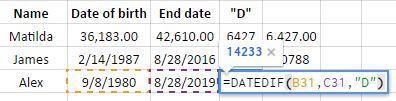Just days

If you want to calculate and age and have it always be current when you open the spreadsheet, consider using the function called TODAY as your end date. TODAY returns the current day.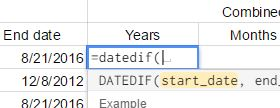## Google Sheets – Calculate age with the DATEDIF function

Calculating age in a spreadsheet can be a painful process using many of the formulas floating around the web today. Dates act strangely in spreadsheets so care must be taken when working with them. Fortunately, calculating age can be done much easier using a single function in Google Sheets called DATEDIF. The DATEDIF function will return the difference between two dates in days, months, or years. Using these units in combination can get you a variety of output options.

Excel also accepts this function, but it is not in its standard list of functions.

## Syntax

`=DATEDIF(start_date,end_date,unit)`

`start_date` Date at which to start the calculation

`end_date` Date at which to end the calculation

`unit` Type of output. Choices are “Y”,”M”,”D”,”YM”,”YD”, or “MD”.

• Y – Number of whole years elapsed between start and end dates
• YM – Number of months elapsed after the number of years shown with the “Y” unit. Will not exceed 11.
• YD – Number of days elapsed after the number of years shown with the “Y” unit. No matter how many days after the last year, starts counting after end of last full year and is never over 364.
• M – Number of whole months elapsed between start and end dates
• MD – Number of days elapsed after the number of months shown with the “M” or “YM” unit. Can’t go higher than 30.
• D – Number of whole days elapsed between start and end dates
Watch the video

This site has a companion YouTube channel that has pretty much, well almost exactly, the same content. If you like this, you'll like that.

## Examples

#### Follow image below for the live Google Sheet with this dataLet’s say that you have three columns of data. As long as you have start and end dates, you are ready to go.Columns for name, start date, and end date

Now add 4 columns to the right for the DATEDIF formula with “Y”, “YM”, “YD”, and a column with them joined together. Remember that all of these are in the live Google Sheet shared above. The Y, YM and MD are used as the “unit” in each respective column. The “Y” column is showing the person’s age in years. The “YM” is showing the number of months that have elapsed since the last year shown. The “MD” column is showing the number of days that have elapsed since the last whole month.Y, YM, and MD units added

There are other types of units as well. This next example uses M and MD. Note that the number of months goes over twelve since the years are not in the formula’s output. The column using MD as the unit is the same calculation as above.M and MD units

This last example uses just the D unit. If you are just using this function to calculate days, it would be easier to just use a subtraction formula for the number of days. Dates in spreadsheets are actually stored as integers so they can easily be added and subtracted. The last column, which is unlabeled, contains a subtraction formula.Just days

If you want to calculate and age and have it always be current when you open the spreadsheet, consider using the function called TODAY as your end date. TODAY returns the current day.